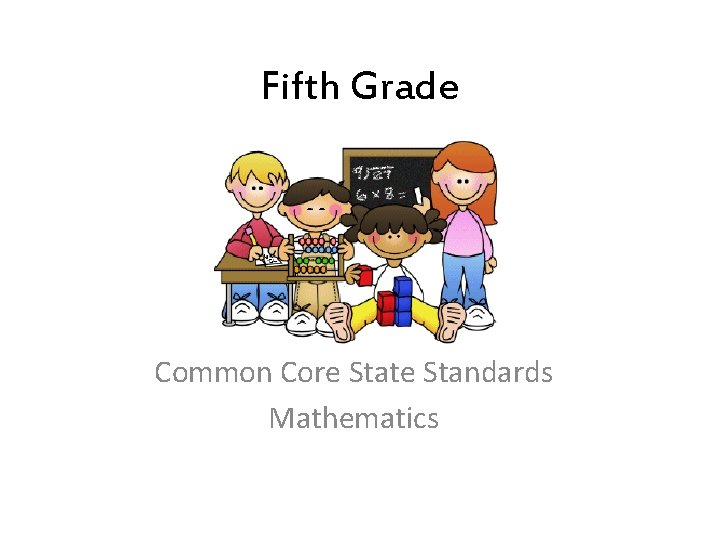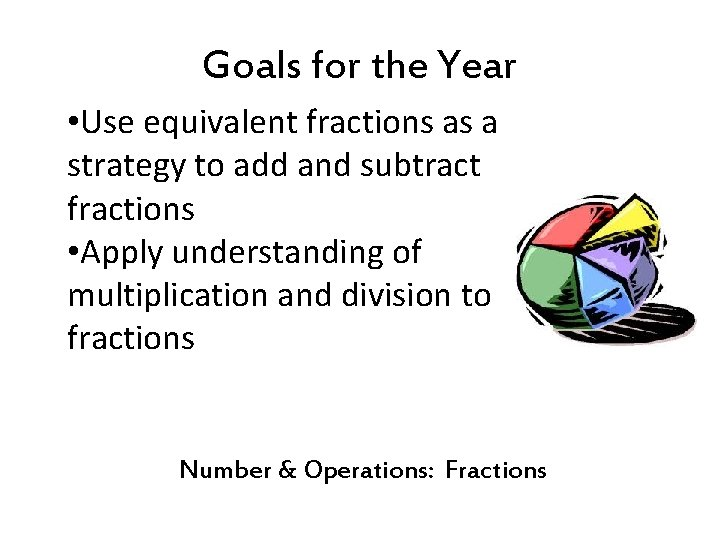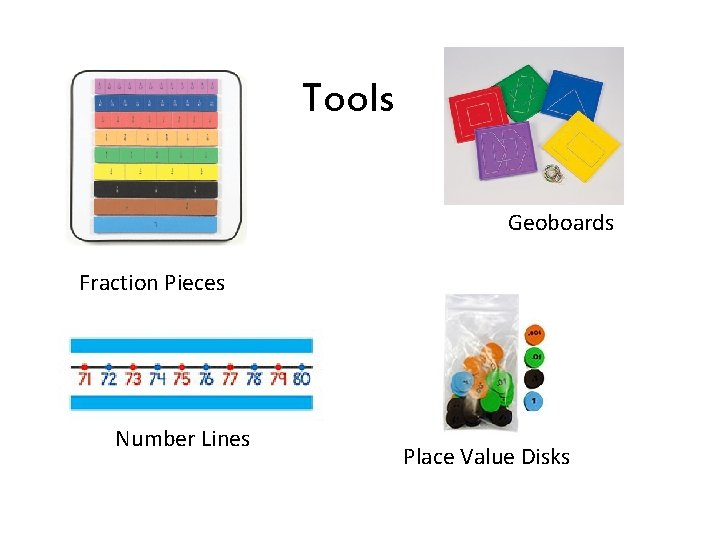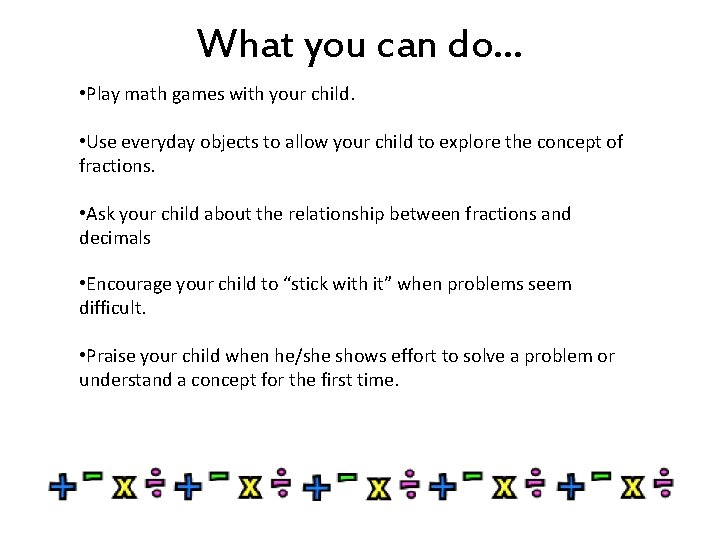# Fifth Grade Common Core State Standards Mathematics Goals

• Slides: 8Fifth Grade Common Core State Standards MathematicsGoals for the Year • Write and interpret numerical expressions • Analyze patterns and relationships Operations & Algebraic ThinkingGoals for the Year • Understand the place value system including decimals and the use of exponents • Perform operations with multi-digit whole numbers and with decimals to the hundredths • Fluently multiply multi-digit whole numbers using the standard algorithm • Find quotients using non-standard algorithms Number & Operations in Base TenGoals for the Year • Use equivalent fractions as a strategy to add and subtract fractions • Apply understanding of multiplication and division to fractions Number & Operations: FractionsGoals for the Year • Convert measurement units within a given measurement system • Represent and interpret data using fractions and line plots • Understand the concept of volume and relate volume to multiplication and division Measurement & DataGoals for the Year • Graph points on the coordinate plane to solve real-world and mathematical problems • Classify two-dimensional figures into categories based on their properties GeometryTools Geoboards Fraction Pieces Number Lines Place Value DisksWhat you can do… • Play math games with your child. • Use everyday objects to allow your child to explore the concept of fractions. • Ask your child about the relationship between fractions and decimals • Encourage your child to “stick with it” when problems seem difficult. • Praise your child when he/she shows effort to solve a problem or understand a concept for the first time.